# Properties of Triangles Chapter Exam

Exam Instructions:

Choose your answers to the questions and click 'Next' to see the next set of questions. You can skip questions if you would like and come back to them later with the yellow "Go To First Skipped Question" button. When you have completed the practice exam, a green submit button will appear. Click it to see your results. Good luck!

### Page 1

#### Question 1 1. Identify x and y in the pictured triangle.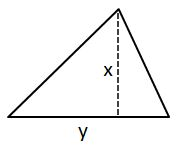#### Question 3 3. In the pictured triangle, what is side B, relative to angle x?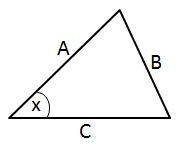#### Question 4 4. What type of line segment is represented by the dotted line in the pictured triangle?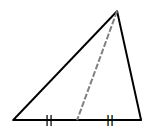#### Question 5 5. If angle a is 60 degrees, what is the measure of angle b?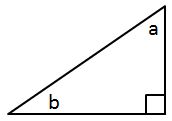### Page 2

#### Question 6 6. Determine if triangle VEZ is similar to triangle MAG.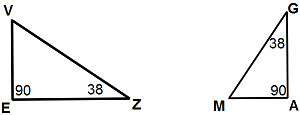#### Question 7 7. What type of triangle is pictured?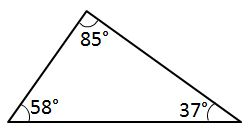#### Question 8 8. If angle a is 68 degrees and angle b is 37 degrees, what is the measure of angle c?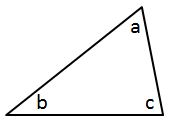#### Question 10 10. What type of triangle is pictured?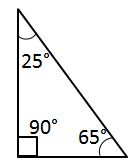### Page 3

#### Question 11 11. In the pictured triangle, which of the following lines is an altitude?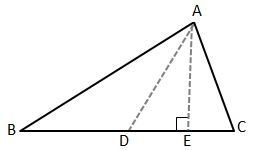#### Question 12 12. What type of line segment is represented by the vertical dotted line in the pictured triangle?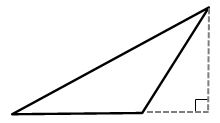#### Question 13 13. What type of triangle is pictured?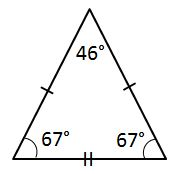#### Question 15 15. Conclude whether triangle BEN is similar to triangle MAT.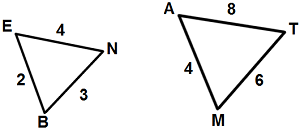### Page 4

#### Question 16 16. In the pictured triangle, identify the altitude(s).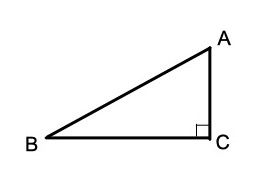#### Question 17 17. Josh claims that these triangles are similar by SSS. Do you agree or disagree with Josh?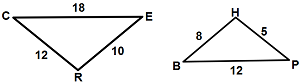#### Question 19 19. What type of line segment is represented by the dotted line in the pictured triangle?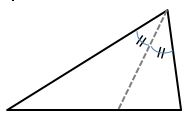### Page 5

#### Question 21 21. Triangle HYV and triangle AYB are similar by the AA similarity theorem. What is the value of x?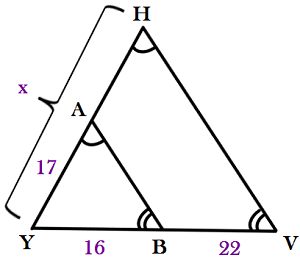#### Question 22 22. Given triangles DAR and KMR. What is the value of y?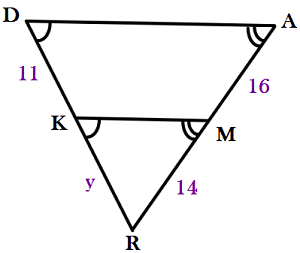#### Question 24 24. If angle a is 80 degrees, what is the measure of angle d?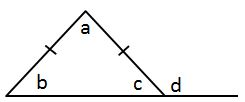#### Question 25 25. If angle a is 66 degrees and angle b is 54 degrees, what is the measure of angle d?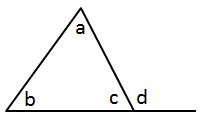### Page 6

#### Question 26 26. If angle a is 30 degrees and angle d is 70 degrees, what is the measure of angle b?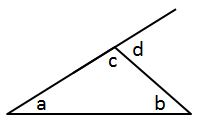#### Question 27 27. Mary claims that the triangles below are similar by AA. Do you agree or disagree with Mary?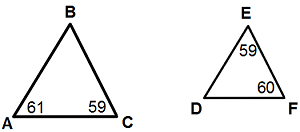#### Properties of Triangles Chapter Exam Instructions

Choose your answers to the questions and click 'Next' to see the next set of questions. You can skip questions if you would like and come back to them later with the yellow "Go To First Skipped Question" button. When you have completed the practice exam, a green submit button will appear. Click it to see your results. Good luck!

Support